Courses

# Previous Year Questions - Arithmetic Progressions Class 10 Notes | EduRev

## Class 10 : Previous Year Questions - Arithmetic Progressions Class 10 Notes | EduRev

The document Previous Year Questions - Arithmetic Progressions Class 10 Notes | EduRev is a part of the Class 10 Course Mathematics (Maths) Class 10.
All you need of Class 10 at this link: Class 10

Short Answer Type Questions

Q.1. If Sn, the sum of the first n terms of an AP is given by Sn = 2n2 2+n, then find its nth term.    [CBSE 2019]
Ans: We have in an AP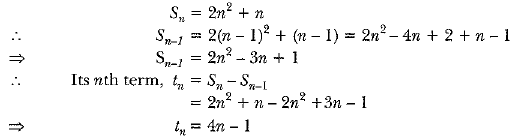Q.2. If the 17th term of an AP exceeds its 10th term by 7, find the common difference.    [CBSE 2019]
Ans:
According to question,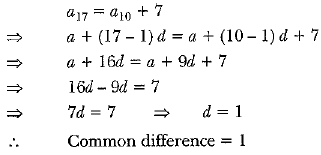Q.3. If in an AP, a = 15, d = -3 and an = 0, then find the value of n.    [CBSE 2019]
Ans: In an AP it is given that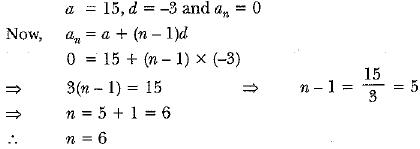Q.4. Which term of the arithmetic progression 3, 15, 27, 39 .... will be 120 more than its 21st term?    [CBSE 2019]
Ans: We have, a = 3 and d = 12
∴ a21 = a + 20d = 3 + 20 x 12 = 243
Let nth term of the given AP be 120 more than its 21st term. Then,
an = 120 + a21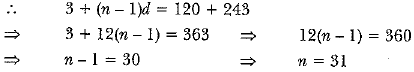Hence, the 31st term of the given AP is 120 more than its 21st term.

Q.5. If Sn the sum of first n terms of an AP is given by Sn = 3n2 - 4n, find the nth term.    [Delhi 2019]
Ans: Given: Sn - 3n2- 4n. Let tn 1be the nth term.
∴ tn = Sn - Sn-1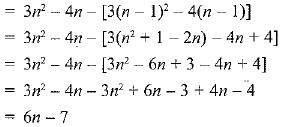Hence, tn = 6n - 7 .

Q.6. In an A.P., if the common difference (d) = - 4 and the seventh term {a7) is 4, then find the first term.    [CBSE 2018]
Ans: Let a = first term,
Given, d=- 4,a7 = 4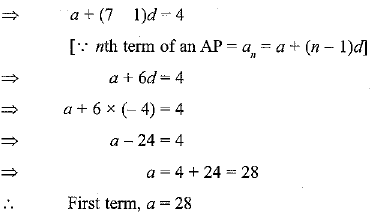Q.7. Find the sum of first 8 multiples of 3.    [CBSE 2018]
Ans:
The first 8 multiples of 3 are 3, 6, 9, 12, 15, 18,21,24.
Here, 2nd term - 1st term = 3rd term - 2nd term
⇒ 6 - 3 = 9 - 6 = 3
Since, common difference is same. Therefore, the above terms are in AP.
Here, a = 3, d = 6 - 3 = 3, n = 8
We know, the sum of n terms of an AP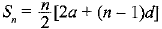where a = first term, d = common difference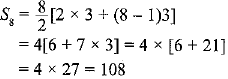Q.8. In an A.P. if the sum of its first n terms is 3n2 + 5n and its kth term is 164, find the value of k.    [CBSE 2018 (C)]
Ans: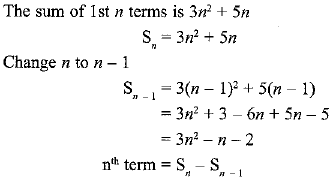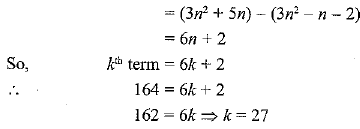Q.9. In an A.P. if the sum of its first n term is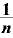and nth term is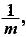then find the sum of its first mn term.    [Delhi 2017]
Ans: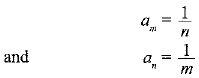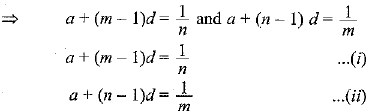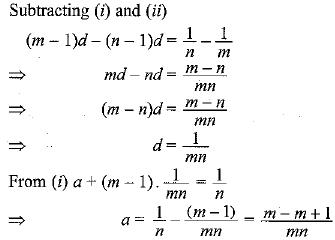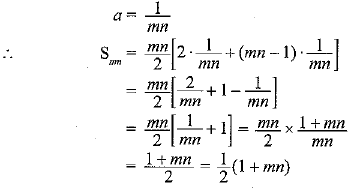Q.10. Write the nth term of the A.P.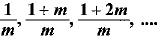[Delhi 2017 (C)]
Ans: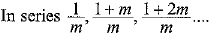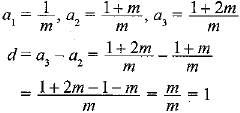nth term of the AP is = a1 + (n - 1)d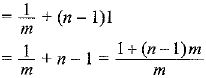Q.11. Find the sum of the following series:
5 + (- 41) + 9 + (- 39) + 13 + (- 37) + 17 +....+ (-5 ) + 8 I + (-3 )    [CBSE (F) 2017]
Ans: The series can be rewritten as,
(5 + 9 + 13 + ... 4- 81) 4- (-41 + (-39) + (-37) + ... + (-5) + (-3))
For the series 5 + 9 + 1 3 + ... 81
a = 5, d = 4 and an = 81
nth term = a + (n - l)d = an
⇒  5 ( n - 1)4 = 81
⇒ 4n = 80
⇒ n = 20
Sum of 20 terms for this series.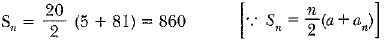...(i)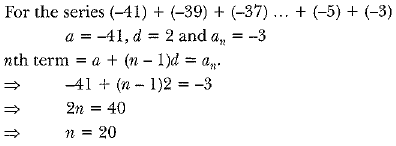Sum of 20 terms for this series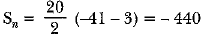...(ii)
By adding (i) and (ii), we get
Sum of series = 860 - 440
= 420

Q.12. If 7 times the 7th term of an AP is equal to 11 times its 11th term, then find its 18th term.    [CBSE (F) 2017]
Ans: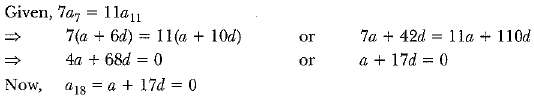Q.13. For what value of n, are the nth terms of two APs 63, 65, 67,... and 3, 10, 17,... equal?    [CBSE(AI) 2017]
Ans: Let nth terms for two given series be an and an
According to question,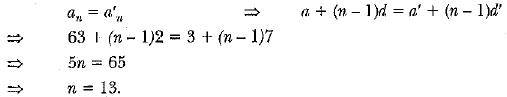Q.14. How many terms of the AP: 9, 17, 25,... must be taken to give a sum of 636?    [NCERT, CBSE (AI) 2017]
Ans: Let sum of n terms be 636.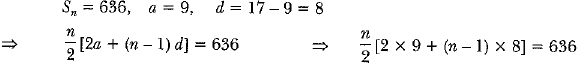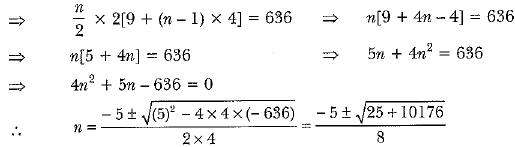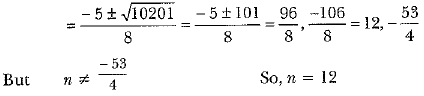Thus, the sum of 12 terms of the given AP is 636.

Q.15. If the sum of the first 7 terms of an AP is 49 and that of 17 terms is 289, find the sum of the first n terms.    [CBSE Delhi 2017]
Ans: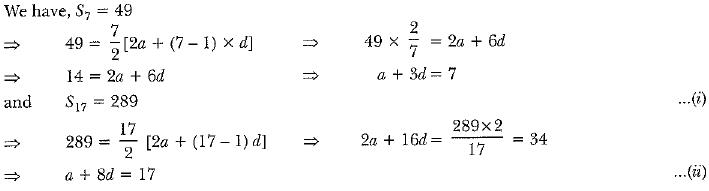Q.16. The first term of an AP is 5, the last term is 45 and the sum is 400. Find the number of terms and the common difference.    [CBSE (AI) 2017]
Ans: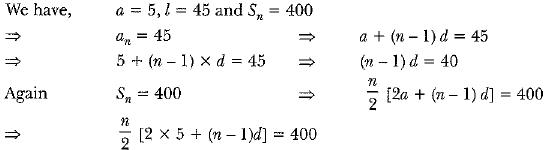...(i)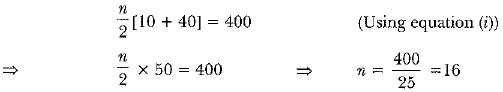Now, putting the value of n in equation (i), we have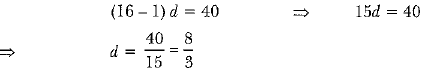Hence, the number of terms is 16 and the common difference is 8/3.

Q.17. What is the common difference of an A.P. in which a21 - a7 = 84?    [AI 2017]
Ans:  Let 'd’ be the common difference of the AP whose first term is 'a'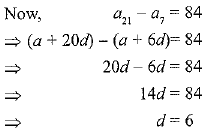Q.18. Find how many integers between 200 and 500 are divisible by 8.    [Delhi 2017]
Ans: Numbers divisible by 8 between 200 and 500 are 208, 216, 224, 232, ..., 496
Since, 216-208 = 224-216
Therefore, the given integers are in A.P.
Let first term (a) = 208,
common difference (d) = 224 -216 = 8,
Number of terms(n) = ?
Last term (an) = 496
We know that, an = a + (n - 1)d
⇒ 496 = 208 + (n - 1)(8)
⇒ 496 - 208 = (n - 1)(8)
⇒ 288 = 8n - 8
⇒ 8n = 288 + 8 = 296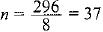Therefore, 37 integers are there between 200 and 500 divisible by 8.

Q.19. Which term of the progression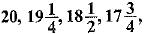... is the first negative terms?    [AI 2017]
Ans: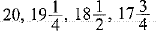In this progression, first term is 20
Also, second term - first term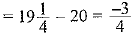third term - second term =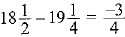fourth term - third term =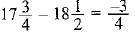∵ Difference between constructive term is constant,
therefore this progression is an AP.
Let nth term be the first negative term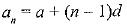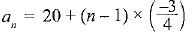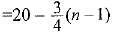Now A.T.Q     an < 0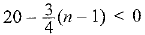⇒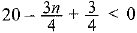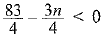⇒  83 - 3n < 0
⇒ 3n > 83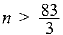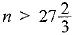⇒ n > 28
[ ∵ n is a natural number]
Thus, 28th term of the given progression is the first negative term.

Q.20. Find the sum of all two-digit natural numbers which are divisible by 4.

[CBSE Delhi 2017 (C)]
Ans: Here a = 12, d = 4, an = 96
The formula is an = a + (n - 1 )d
Therefore 96 = 12 + (n - 1) x 4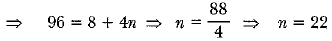Apply the formula for sum,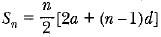Hence, S22 = 11[24 + 21 x 4] = 11 [24 + 84]
= 11 x 108 = 1188.

Q.21. The ratio of the sum of first n terms of two different AP’s is (7n + 1) : (4n + 27), find the ratio of their mth terms.    [CBSE (AI) 2016]
Ans: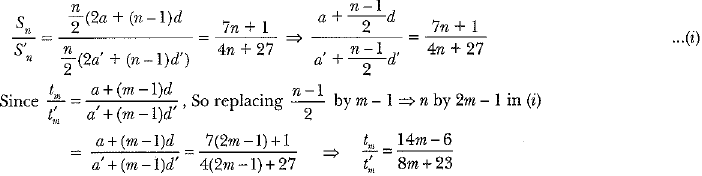Q.22. Find the 9th term from the end (towards the first term) of the AP 5, 9, 13,..., 185.    [CBSE (Delhi) 2016]
Ans: l = 185, d = 4
l9 = l - (n - 1) d = 185 - 8 x 4 = 153

Q.23. How many terms of the AP 18, 16, 14,.... be taken so that their sum is zero?    [CBSE Delhi 2016]
Ans: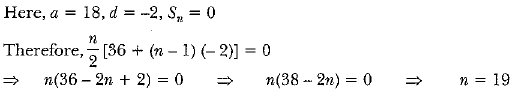Q.24. The 4th term of an AP is zero. Prove that the 25th term of the AP is three times its 11th term.    [CBSE (Al) 2016]
Ans: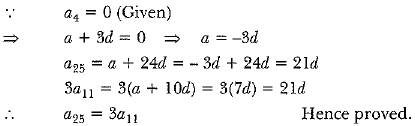Q.25. If the ratio of the sum of the first m and n terms of an AP is m2: n2, show that the ratio of its mth and nth terms is (2m - 1) : (2n - 1).    [CBSE (F) 2016]
Ans: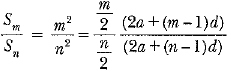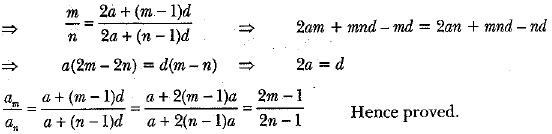Q.26. The first term of an A.P. is 5, the last term is 45 and the sum of all its term is 400. Find the number of terms and the common difference of the A.P. [AI 2017]
Ans: Let 'a' be the first term and 'd' be the common difference of the given AP.
Let an be the last term and Sn be the sum of n terms
so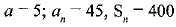We need to find the values of 'n' and 'd'
We know that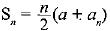...(i)
Substituting the values in (i)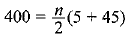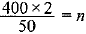⇒ n = 16
Also, an = a + (n - 1)d
⇒ 45 = 5 + (16 - 1 )d
⇒ 45 - 5 - 15d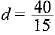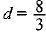Hence, there are 16 terms and the common difference is 8/3.

Long Answer Type Questions

Q.1. The first term of an AP is 3, the last term is 83 and the sum of all its terms is 903. Find the number of terms and the common difference of the AP.

[CBSE 2019 ]
Ans: We have an AP, in which
a = 3, last term, an = l = 83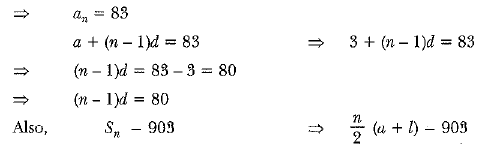...(i)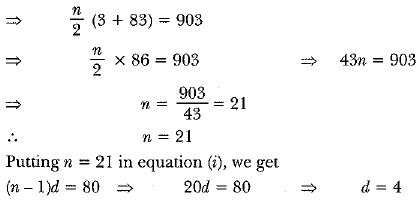No. of terms =21 and common deference = 4

Q.2. Which term of the Arithmetic Progression - 7, - 12 , - 17 , - 22 , ... will be - 82. Is - 100 any term of the A.P.? Give reason for your answer.    [CBSE, Allahabad 2019]
Ans: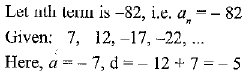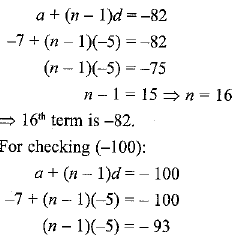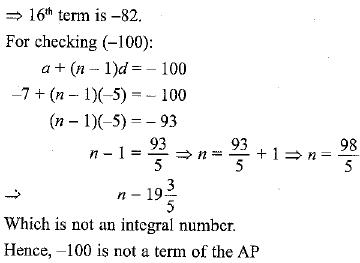Q.3. The sum of four consecutive numbers in an AP is 32 and the ratio of the product of the first and the last term to the product of two middle terms is 7: 15. Find the numbers.    [CBSE 2018]
Ans:
Let the four consecutive numbers of the AP are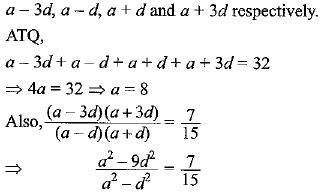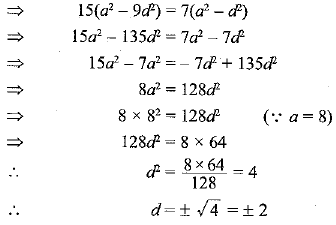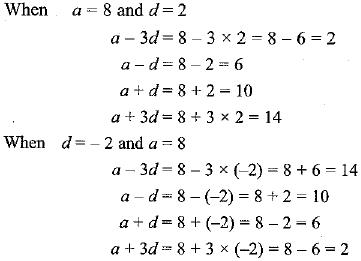Therefore, four consecutive terms of the AP’s are 2, 6, 10, 14 or 14, 10, 6, 2 respectively.

Q.4. If the ratio of the 11th term of an AP to its 18th term is 2 : 3, find the ratio of the sum of the first five terms to the sum of its first 10 terms.    [CBSE Delhi 2017]
Ans: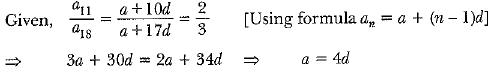...(i)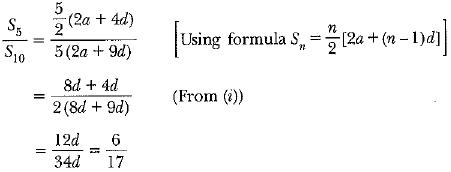Hence S5 : S10 = 6 : 17.

Q.5. If the ratio of the sum of the first n terms of two A.P.s is (7n + 1) : (4n + 27), then find the ratio of their 9th terms.    [AI 2017]
Ans:
Let a1, a2 be the first terms and d1, d2 be the common differences of the two given AP’s
Then the sums of their n terms is given by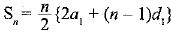and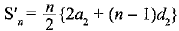A.T.Q.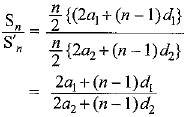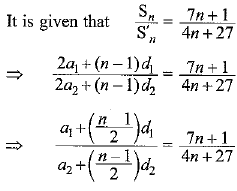Q.6. Find the sum of the first 24 terms of an A.P. whose nth term is given by an = 3 + 2n.    [CBSE AI 2017 (C)]
Ans:
nth term of the given AP is
an = 3 + 2n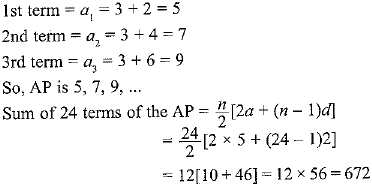Q.7. In an AP of 50 terms, the sum of the first 10 terms is 210 and the sum of its last 15 terms is 2565. Find the AP.    [CBSE  (F) 2017]
Ans:
Let 'a' be the first term and 'd' the common difference.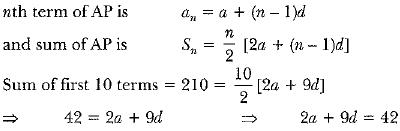...(i)
15th term from the last = (50-15 + l)th =36th term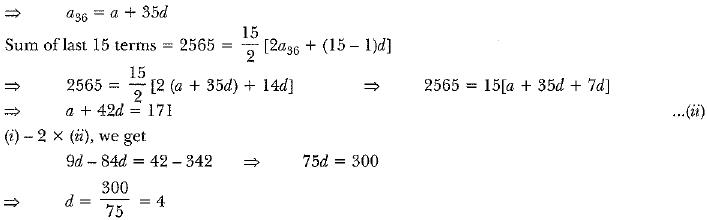Putting the value of d in (ii)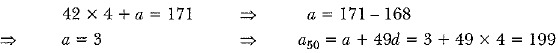So, the AP formed is 3, 7, 11, 1 5 , ..... and 199.

Q.8. Divide 56 into four parts which are in AP such that the ratio of product of extremes to the product of means is 5 : 6.    [CBSE (F) 2016]
Ans: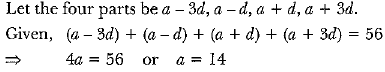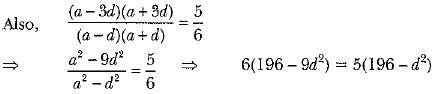[∵ a = 14]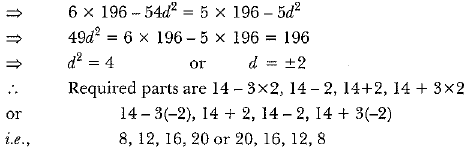Q.9. A thief runs with a uniform speed of 100 m/minute. After one minute a policeman runs after the thief to catch him. He goes with a speed of 100 m/minute in the first minute and increases his speed by 10 m/minute every succeeding minute. After how many minutes the policeman will catch the thief?    [CBSE (Delhi) 2016]
Ans: Let total time be n minutes
Total distance covered by thief = 100 n metres
Total distance covered by policeman = 100 + 110 + 120 + ... + (n - 1) terms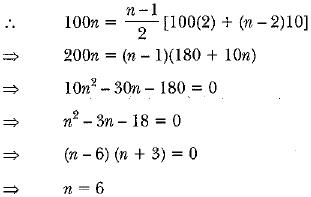Policeman took (n - 1) = ( 6 - 1 ) = 5 minutes to catch the thief.

Q.10. The houses in a row are numbered consecutively from 1 to 49. Show that there exists a value of X such that the sum of numbers of houses preceding the house numbered X is equal to the sum of the numbers of houses following X. Find the value of X.    [CBSE 2016]
Ans: The numbers of houses are 1, 2, 3, 4 ......... 49.
The numbers of the houses are in AP, where a = 1 and d = 1
Sum of n terms of an AP =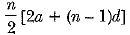Let Xth number house be the required house.
Sum of number of houses preceding Xth house is equal to SX-1 i.e.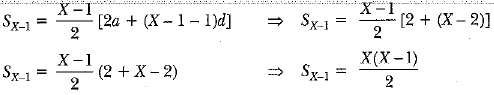The sum of numbers of houses following Xth house is equal to S49 - SX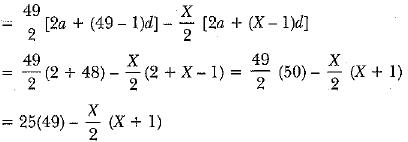Now, we are given that sum of number of houses before X is equal to the sum of the number of houses after X.
i.e., SX-1 = S49 - Sx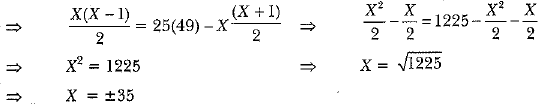Since number of houses is positive integer, ∴ X = 35

Offer running on EduRev: Apply code STAYHOME200 to get INR 200 off on our premium plan EduRev Infinity!

## Mathematics (Maths) Class 10

51 videos|346 docs|103 tests

,

,

,

,

,

,

,

,

,

,

,

,

,

,

,

,

,

,

,

,

,

;